In the previous course, we explained that when the modulator is a continuous variable, we use whether the interaction terms of the independent variable and the modulator are significant to the path coefficient of the dependent variable to determine whether the moderating effect is significant. However, when the moderator is categorical, the interaction term method is completely inapplicable, in which case it is necessary to use AMOS categorical moderator multi-group analysis. Let’s explain it through practical cases.

The following is the data of X and Y indicators for 190 patients, M is sex, and I wanted to study the moderating effect of gender on the influence of X on Y (Figure 1)

Figure 1

AMOS classification moderator multi-cohort analysis procedure

(1) Open the amos and draw the structural model diagram (Figure 2)

Figure 2

(2) Create a new group, double-click the blank space in the figure below, and click “New” (Figure 3)

Figure 3

(3) After importing the data, click Grouping Variable, select Gender T, and click OK (Figure 4)

Figure 4

(4) Click Group Value again, select category 0, and click OK (Figure 5)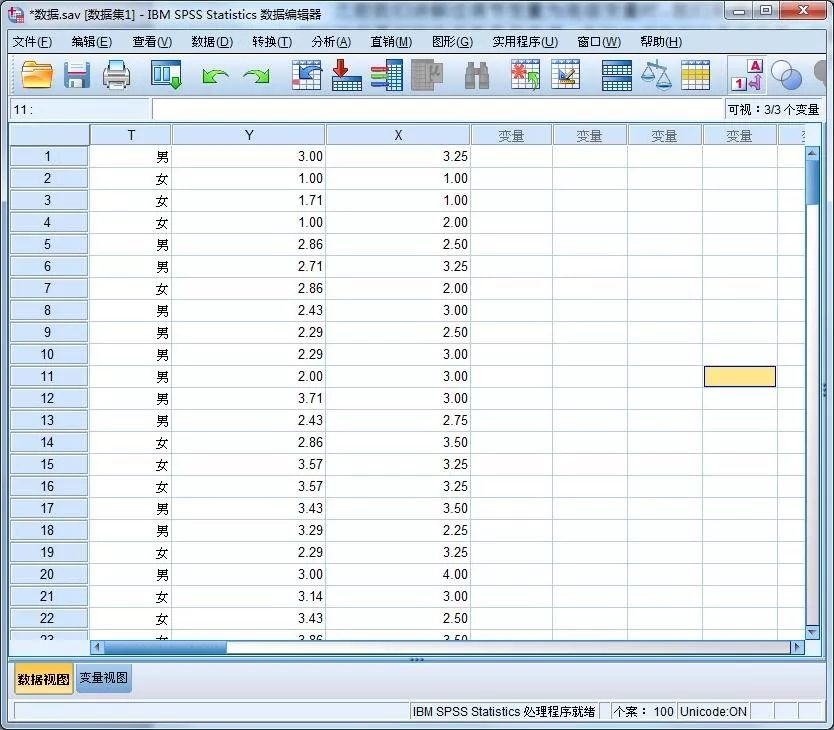Figure 5

(5) Use the same method to set Group number2, that is, after group 2 is set to category 1 of the categorical variable, as shown in Figure 6

Figure 6

(6) Drag the data into the variable box (Figure 7)

Figure 7

(7) Click Multiple-Group Analysis to set it in Figure 8 below

Figure 8

(8) Set the residual item and check the output option setting (Figure 9)

Figure 9

(9) Click to calculate and analyze the results. Click Model Fit under View Text to view the CMIN and DF values of the Unconstrained model and the Structural weights model (Figure 10), and calculate the difference values, △CMIN=13.907, △DF=1, respectively

Figure 10

(10) Calculate the significance level in Excel, apply the CHIDIST function (Figure 11), substitute the above, △CMIN and △DF to calculate the significance level is 0.0001 < 0.05, it is concluded that the modulating effect of M in X →Y is significant.

Figure 11

After obtaining the significant adjustment effect, it is necessary to further analyze the difference between the two categories of the adjustment effect, here you need to return to the output interface settings, check Critical ratios for differences and then perform the calculation (Figure 12)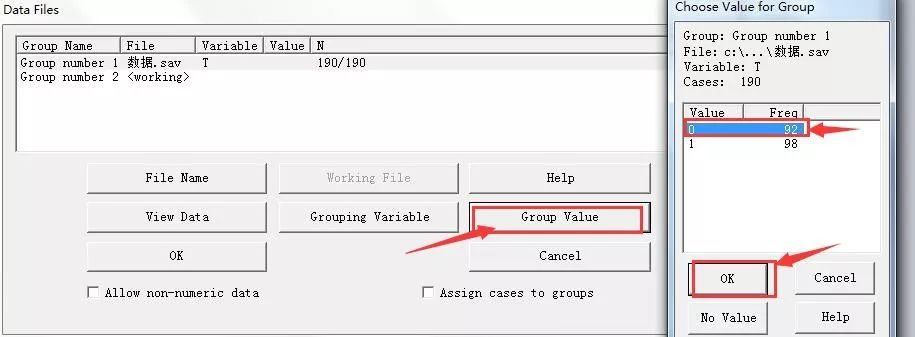Figure 12

Finally, click Pairwise Parameter Comparisons under View Text, view the value as shown in the figure (Figure 13), and see that the b1_1 and b1_2, that is, the values of women and men are -3.799, and their absolute value size is greater than 1.96, which concludes that there are significant differences in the regulatory effect between men and women, further indicating that the regulatory effect of gender is significant.

Figure 13

That’s all for this issue, more AMOS statistical analysis courses will be updated in the future, please pay attention! We will continue to launch more practical biomedical statistics courses, covering SPSS, Meta, Stata, GraphPad, SAS, R, NoteExpress, EndNote, Revman and other data statistical analysis software and statistical methods! Stay tuned!

Xinghuakai Biomedical Statistics Customer Service QQ3305200052

【Apricot Blossom Biomedical Statistics】Related Articles:

·

Application of Least Squares Regression Model in Biomedical Statistics(2)

Application of Least Squares Regression in Biomedical Statistical Analysis (1)

Analysis of Covariance for SPSS ANOVA (2) Covariance Analysis Operation

SPSS ANOVA (1) Preconditions for covariance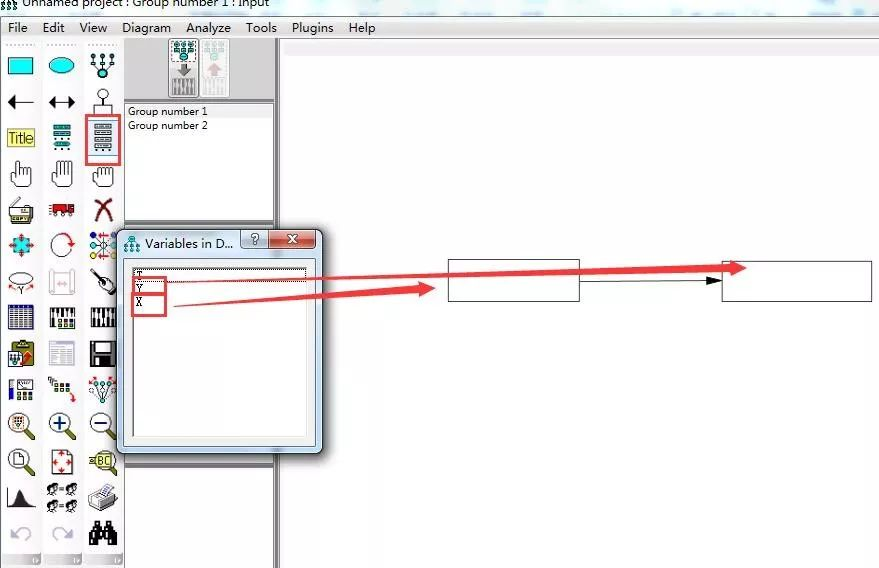Operation and application of SPSS discriminant analysis (2) Stepwise discriminant analysis

Operation and application of SPSS discriminant analysis (1) General discriminant analysis

SPSS clustering analysis (K-means clustering) software operation results interpretation

﻿·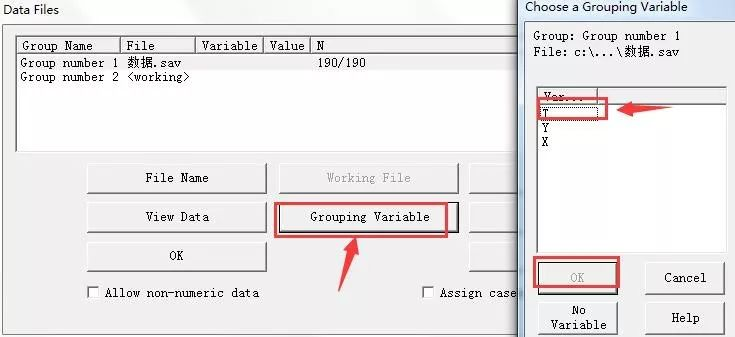Software operation and interpretation of results of SPSS cluster analysis (R-type clustering).

Software operation and interpretation of results of SPSS clustering

Detailed application and operation of SPSS curve regression analysis

Multivariate ANOVA (3) Graphpad simple effect line chart

Multivariate ANOVA for SPSS ANOVA (2) (Simple Effect)

Multivariate ANOVA for SPSS ANOVA (1)

Application of SPSS virtual linear regression analysis in statistical analysis of questionnaire data

SPSS conducts practical operation and analysis of mediation effect test

AMOS structural equation tutorial, Detailed interpretation of SPSS moderating effect analysis operations and results

Application and operation of SPSS mixed linear model in biomedical statistics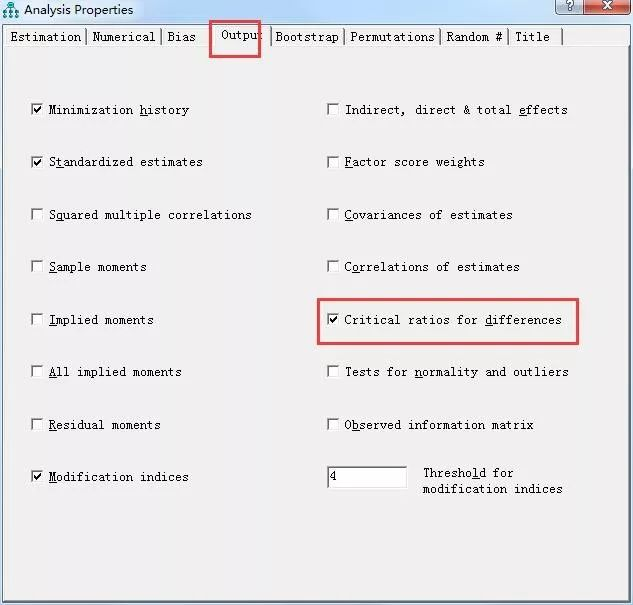Application of SPSS disordered logistic regression in biomedical statistical analysis

AMOS processed mediating effect analysis in biomedical questionnaires

AMOS conducts validation factor analysis for validity analysis of questionnaire analysis

SPSS regulated regression analysis is applied in biomedical statistical analysis

Application of SPSS repeated-measure ANOVA in biomedicine

SPSS compares differences between groups and their pairsSPSS-independent sample t-test versus SPSS one-way ANOVA

GraphPad Prism plots the ROC curve for specific operation steps

SPSS performs a normal distribution test for continuous data

The specific operation of SPSS ordered logistic regression

COX risk ratio regression for SPSS survival analysis in biomedical statistics

Application of SPSS binary logistic regression in biomedical statistical analysis

Medcalc software accurately compares the diagnostic accuracy of multiple indicators

Application operation and analysis of SPSS multiple linear regression

How SPSS and GraphPad compare within- and between-group differences

AMOS Structural Equations for Medical Questionnaire Scale Analysis Tutorial

Application of SPSS survival analysis in biomedical statistics

GraphPad Prism statistical plot histogram, box plot

In SPSS, the detailed operation and interpretation of the results of exploration factor analysis on the questionnaire data are performed

Project analysis of SPSS questionnaire data statistical analysis

Excel performs operations on linear regression model analysis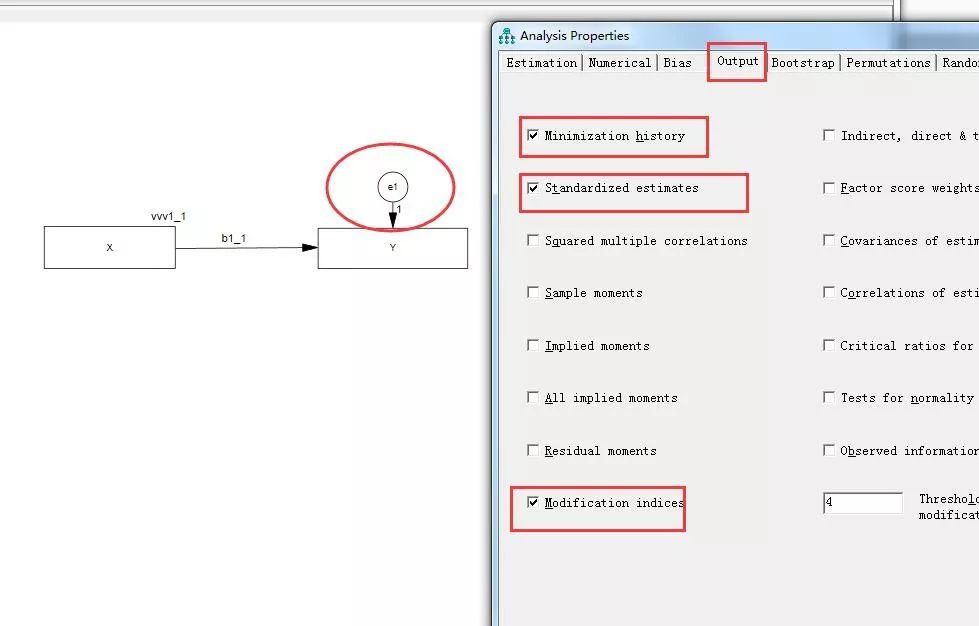Parametric and non-parametric test methods commonly used in SPSS

Operation and application of ROC curve in SPSS

Read how R can achieve stepwise regression analysis

How to draw scatterplots and boxplots in R?

Introduction to the Variable data variable types in SPSS

Correlation analysis in SPSS, correlation coefficient implications

Read SPSS one-way ANOVA and ANOVA in one article

The calculation method and specific significance of the coefficient R of linear regression determination

The whole process of medical and biostatistics

Hypothesis testing in biological and medical statistics

GraphPad Prism Detailed tutorial on plotting survival curves

·

·

·

·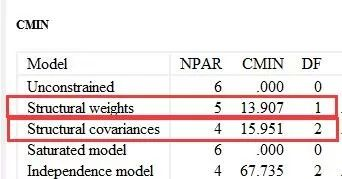·

·

·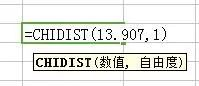·

·

·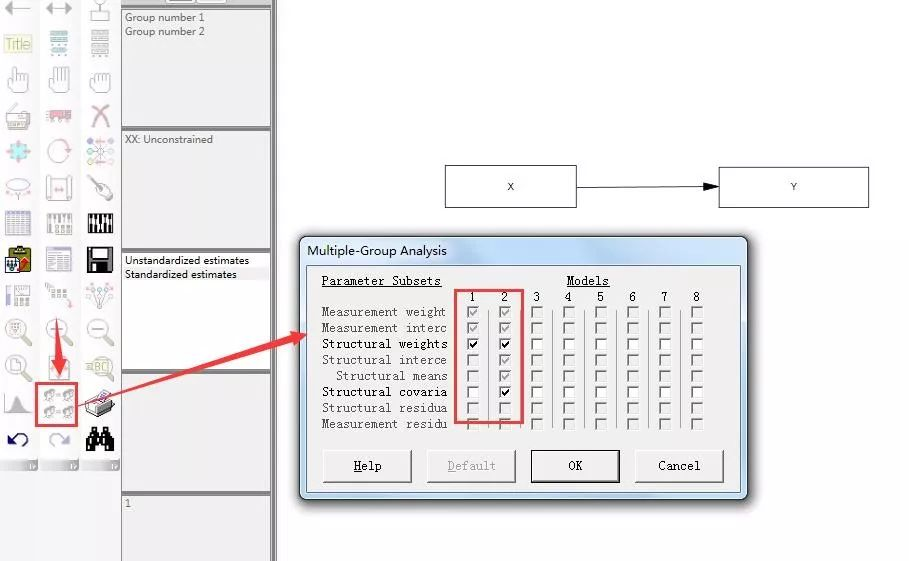··

·

·

·

·

·

·

·

·

·

·

·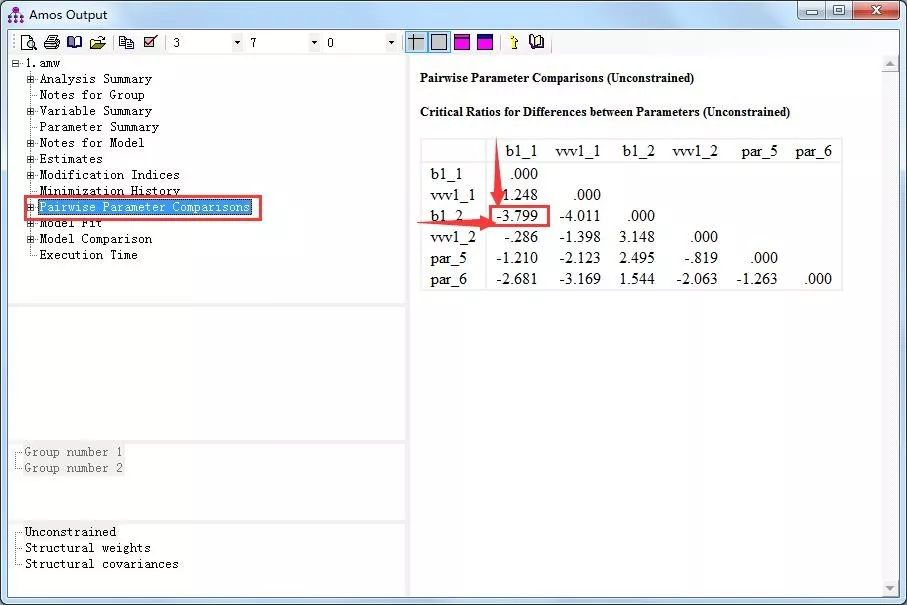·

·

·

·

·

·

·

·

·

·

·

·

·

·

·

·

·

·

·

·

﻿·

﻿·

﻿·

﻿·